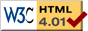Version 0.2.2

# S-Lang Grace Plotting Module Tutorial

To illustrate the use of `grace-module` from the S-Lang interpreter, the examples on this page will be given in the context of an interactive slsh session. Of course the use of the module is not limited to slsh; the examples could just as easily be given in the context of the jed editor or any other application that embeds the S-Lang interpreter.

In order to make use of the module it must first be loaded into the interpreter. This may be accomplished using the require statement, e.g., Then the grace_new function should be used to create an instance of the Grace plotting application: Assuming Grace is properly installed and that you are working in an X-Windows environment, this will bring up a Grace GUI and assign an object representing it to the variable g. If running outside X windows, Grace will run in batch mode.

A simple graph may be made using the plot method. For example, to plot the sine of x for various values of x, use

The oplot method may be used to add additional curves to the graph. For example, The resulting graph is shown below.The fact that the second curve was plotted in a different color is a feature of the module: the color index is automatically incremented with each subsequent call to the oplot method. If a specific color is desired, then the ``color-qualifier'' may be used: Keep in mind that qualifiers are separated from function parameters by a semi-colon --- not a comma! The plot and oplot methods take many different qualifiers that affect the look of the plot. For example, the line qualifier controls the linestyle and the width qualifier controls the line width: Symbols may also be plotting at each of the data points. For this, the sym qualifier is used to specify the symbol to be drawn, e.g.,Note that the above figure contains not only the symbols, but also has a line connecting the points. The line=0 qualifier may be used to to turn off the line: If it is desired to have symbols that are filled, the symfill qualifier may be used, as illustrated below.

Most of the Grace plot object methods display a usage message when called with no arguments. For example, Note that simply attempting to call the plot method via will not result in it being called. This is because g.plot is a reference to the underlying function. To actually call the function, you must add the parentheses. To access the online help for a particular Grace object method, use, e.g., As the help and usage forms indicate, if a third argument is passed to the plot method, that argument will be interpreted as an error bar. For example,Error bars in X can also be plotted. To force the third argument to be draw as an X error bar, use the type qualifier set to the value "xydx", i.e., For errorbars in both X and Y, use, e.g., In addition to the plot and oplot methods, histogram plots may be made using the hplot and ohplot methods. The following example assumes that you have installed the histogram and GNU Scientific Library modules (both just an ``apt-get install'' away on Debian Linux). Assuming this to be the case, load the modules: Now use the gsl-module to produce some random data and bin the data into a histogram: Here the gsl-module ran_gaussian function was used to generate 500 Gaussian distributed random numbers with a sigma of 2. Those points were binned into a ``counts'' histogram with a bin-size of 0.2. Now plot the histogram using the hplot method: Again, qualifiers can be used to get a fancier looking plotIt is interesting to compare the empirical distribution of the data with that of the theoretical Gaussian distribution. The expected number of data points in each bin may be computed and the plotted using:Another way of comparing the expected values with the ``observed'' data is through a plot of the residuals. The multi method may be used to add additional graphs to the plot. Here we want to create two graphs stacked upon one another with a size ratio of 3 to 1: The focus method is used to specify the active graph that will be the target of the plotting commands. To plot the residuals in the second graph use:A more interesting plot is one with color-coded residuals. Here, the idea is to color the residuals that are positive in one color, and those that are negative in another. This will be accomplished by making two calls to the hplot functions:Now let's add some labels and a legend to the plot:And don't forget to save it! In the examples presented here, the data to be plotted were generated on the fly. Often the data will come from an external source, such a binary or text file. Modules are available for interacting with HDF5 and FITS binary files, as well as various databases. Text or so-called ascii files may be read using the S-Lang `readascii` function, e.g., The readascii function is very flexible and supports a number of qualifiers. Use for additional information about its use.

This page was last updated Sep 8, 2007 by John E. Davis. To comment on it or the material presented here, send email to jed at jedsoft org.